Multiprocess
Multilevel Modeling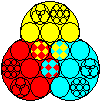aML

What does "aML" stand for?

Q: What does "aML" stand for?
A: Initially, aML was an abbreviation of "applied Maximum Likelihood," because we were developing a general-purpose maximum likelihood optimization package. (All optimization in aML uses Full Information Maximum Likelihood, or FIML.) We subsequently realized that many users saw aML primarily as a powerful package for estimating multilevel models, and only secondarily as a tool for addressing endogeneity and other problems that require multiprocess modeling. As aML evolved, we therefore started thinking of aML more and more as "applied MultiLevel."

Q: How does aML number data levels?
A:
 The most aggregated data unit is level 1 in aML. It represents the highest level. Level 1 is thus the unit of observation. There may be zero or more level 2 units ("branches") in each level 1 unit, zero or more level 3 branches in each level 2 branch, et cetera. See the figure. Lower level branches are often called "replications," and outcomes in those branches "repeatedly observed." Careful: other multilevel software packages may assign level 1 to the most disaggregated level, i.e., they count levels in reverse order. The order has no practical implications. Back to top.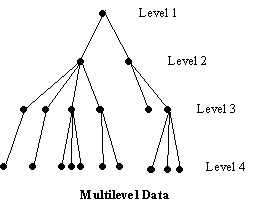Q: Does aML support the Cox proportional hazard model?
A: No, it does not. In practice, however, this is not a limitation. The Cox model differs from other hazard models in that it does not require you to specify the functional form of the duration dependency (Weibull, Gompertz, etc.) This is great, because there is no risk of picking an incorrect functional form. There are also downsides: Cox's estimation algorithm (partial maximum likelihood) is less efficient that full maximum likelihood, so that you get standard errors that are larger than you would get with a parameterized duration dependency. In addition, the Cox model allows no insight into the duration pattern, i.e., how the hazard varies over time.

aML requires a piecewise-linear duration dependency (baseline hazard). In other words, the user specifies as many nodes (bend points) as desired, and aML estimates the slopes that best fit the data. Unlike Weibull, Gompertz, etc, this barely restricts the functional form of the duration dependency; it is semi-parametric and will adjust to any pattern in the data. Consider the figure below which shows the baseline log-hazard of divorce as a function of marriage duration, i.e., the marriage duration dependency.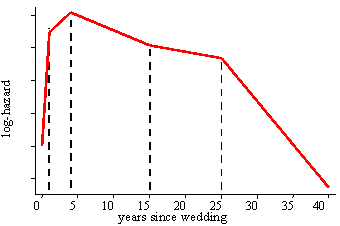Piecewise-Linear Log-Hazard of Divorce

A neat extension, unique to aML, is that it allows for multiple duration dependencies. For example, the hazard of divorce may depend not only on the marriage duration, but also on husband's age, wife's age, calendar time, and possibly more concepts of time. Each may be specified as a piecewise-linear (spline) pattern with its own nodes and slopes. Since all duration dependencies are piecewise-linear, their sum is again piecewise-linear.

Q: Does aML support multinomial logit/probit models?
A: Yes, it does. Version 2 supports both multinomial logit and multinomial probit models. (Version 1 could estimate multinomial probit models, but in a very cumbersome manner.) There is no limitation on the number of categories the multinomial logit can distinguish. The multinomial probit supports up to four categories (three plus one omitted category).

Q: How do I compute predicted multinomial probit probabilities?
A: aML is primarily a package for estimating models, not (yet) for simulating. It currently does not feature a capability for writing out predicted outcomes, probabilities, etc. However, you could compute predicted multinomial probit (and other) probabilities in another software package. Click here for a discussion.

Q: What exactly is a spline?
A: A spline is a piecewise-linear transformation of some variable. Splines play two important roles in aML. First, all duration dependencies of hazard models must be specified as splines. The log-hazard is then piecewise-linear or piecewise-Gompertz. The hazard thus increases and decreases as time ticks on, depending on the signs of the slope coefficients, as illustrated in the figure above. Duration splines start life with a "define spline" statement. Second, you may split up the range of any variable into segments, and estimate piecewise-linear effects. This is typically done by transforming the variable using the spline-transformation as part of a "define regressor set" statement. A spline with n nodes (bend points) generates n+1 segments and thus n+1 slopes.

Mathematically, there are several equivalent ways to spline a variable. In aML, the definition is such that the coefficients may be directly interpreted as slopes on particular segments of the variable (as opposed to the difference between the current segment's slope and the preceding one, which would result from a "marginal" spline transformation.)

Q: What exactly is an observation?
A: An observation is a set of observed outcomes and explanatory variables that is statistically independent from other sets. Two annual wage records of the same person are statistically dependent, need to be modeled jointly, and are thus part of the same observation. Wage records of another person (who is not related to the first person) are independent from those of the first person, and thus part of another observation. An observation in aML always corresponds to the highest level, the most aggregated level, level 1. There may be multiple ASCII records, possibly in multiple ASCII files, pertaining to one observation. Raw2aml and aML will know that they are part of the same observation because they all have the same ID.

Q: What exactly is a regressor set?
A: A regressor set is a vector of variables, say X, which form a regression equation,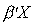. The coefficient vector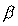may be estimated (or assigned a fixed value). The variables are typically just variables in the data set, but may be formed as transformations or interactions of variables. The resulting regression equation may be used in any model. Think of a regressor set as a, but realize that the elements of X may be expressions involving zero or more variables. The variables may be at any level.

Q: What exactly is a residual draw?
A: Residuals are unobserved and their values for specific outcomes almost always unknown. While we do not know their values, we do know which residuals are correlated with each other (multiprocess settings) and which are equal to each other, i.e., shared by multiple outcomes (multilevel settings). Correlated or shared residuals are realizations of the same draw from a distribution. It is essential to correctly specify which residuals result from the same draw. This is done with the "draw" feature in residual specifications. Draw specifications are a nifty way of telling the program which residuals are correlated and which are independent. See page 333-340 of the Reference Manual for a detailed discussion.

Q: Exactly how are multivariate distributions integrated-out?
A: Where no closed form solution exists, aML allows numerical integration of normally distributed residuals. As explained in the User's Guide (pages 103-5 and 274-5 in Version 1, pages 130-2 and 305-7 in Version 2), the numerical approximation is based on Gauss-Hermite quadrature. The user may choose the number of integration points; the higher the number, the more accurate the approximation. The manual illustrates points of support and their weights for a univariate example and only briefly describes the algorithm for multivariate distributions:
The points are chosen using Gauss-Hermite Quadrature. Suppose you specify a normal distribution with k dimensions and n integration points in each dimension. aML first computes n standardized points which approximate a univariate standard normal distribution. [...] This method is extended to handle k-variate integration by selecting all nk possibilities of k draws out of n points (with replacement). The appropriate weight for each set is the product of k individual (univariate) weights. These nk sets of points and weights approximate a k-variate standard normal. In order to approximate other k-variate normals (with zero means), aML premultiplies each set of k draws by the Cholesky decomposition of the covariance matrix of the distribution. The marginal likelihood is computed as the properly weighted accumulation of nk conditional likelihoods.
The following figures illustrate this approach. Consider numerical integration of a bivariate distribution with six points in each dimension (k=2, n=6). Auxiliary program points, which comes with aML, tells us that the Gauss-Hermite Quadrature support points and weights that approximate a univariate N(0,1) distribution are:

```             points       weights
1   -3.324257E+00  2.555784E-03
2   -1.889176E+00  8.861575E-02
3   -6.167066E-01  4.088285E-01
4    6.167066E-01  4.088285E-01
5    1.889176E+00  8.861575E-02
6    3.324257E+00  2.555784E-03
```

The first figure below shows the support points of two univariate normal distributions, approximated by six points each. The size of the circles is indicative of the weights.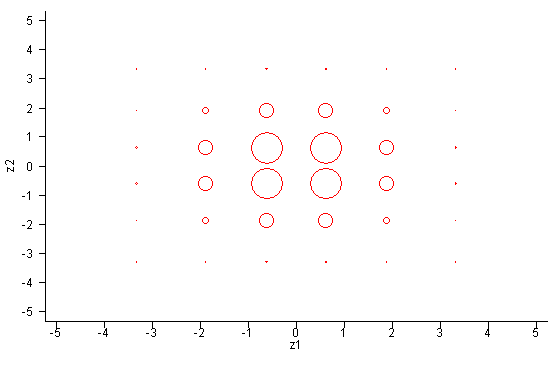Now let's consider a bivariate distribution with covariance matrix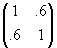. Its Cholesky decomposition is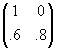. Premultiplying each pair of univariate support points by this Cholesky matrix yields support points of the correlated bivariate distribution: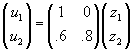. The figure below graphs these support points.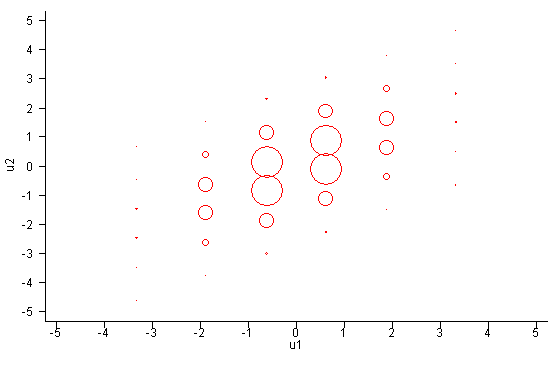One perhaps undesirable implication now becomes clear. Even though the bivariate distribution is symmetric in the sense that residuals may be reversed without consequence, the support points are not symmetric. Residual u1 is sampled with six unique values; residual u2 with 36 unique values. This implies that reversal of residuals in model statements will slightly affect the results of estimation. Both are approximations and neither is better than the other. The differences will become smaller as the approximation improves, that is, as the number of support points increases.

Q: ERROR: the maximum dimension of scratch arrays is exceeded -- What to do?
A: aML keeps lots of scratch arrays in memory. Some of these, especially covariance matrices of residuals and their derivatives, can get quite large when there are very many outcomes per observation. By default, aML allocates 16MB for such scratch arrays, which is typically sufficient for up to approximately 600 outcomes per observation. In rare cases, this is exceeded.

If you receive this error message, you should first make sure that the unit of observation is correct. Do you really have huge numbers of outcomes per observation, or is there perhaps something wrong with the IDs that tie outcomes torgether into observations? Check the data summary information (.sum) file, produced by raw2aml, for number of observations and number of branches within observations. Also check the aML output (.out) file for number of observations and outcomes in your model. Is there really something unobserved that is common to all those outcomes in an observation? If not, re-define your ID variable to refer to a smaller unit.

If the data are correctly structured, try running a version of aML that allocates more memory to scratch arrays: bigaml (64MB) or hugeaml (256MB). Run "bigaml" or "hugeaml" in the same way as you would run "aml". These versions support roughly 1,200 to 2,400 outcomes per observation. These larger versions are located in the same directory as your current aml (or aml.exe) executable file.

Please be aware that computations involving very large covariance matrices can be very slow. If nothing appears to happen for a while, try a smaller sample (option obs=...;) just to make sure that the bottleneck is CPU resources.

If even hugeaml results in the above error message, consider ways to reduce the size of your observations. Can you perhaps ignore unobserved heterogeneity at the top level, or can it be replaced by something observed and captured through (dummy) variables? ("Option huber" generates Huber-corrected standard errors, also known as robust standard errors, which offer some protection against clustering of observations.) Or consider keeping a random subset of outcomes; if you cannot identify heterogeneity with, say, 1,000 outcomes per observation, it is probably not there, and you may re-define observations into smaller units.

Finally, beware of aML's default estimates of standard errors. With very many outcomes per observation, your data may end up having very few observations. Standard errors are the square roots of diagonal elements of the parameter estimates' covariance matrix, computed as the inverse of the matrix of second derivatives (Hessian matrix). By default, that Hessian matrix is approximated as minus the sum over observations of the outerproduct of first derivatives (BHHH method). The BHHH approximation is asymptotically correct, but may be poor in finite samples. "Option numerical standard errors" circumvents the BHHH approximation by computing the Hessian as numerical derivatives of (analytical) first derivatives. Use that option to obtain more precise estimates of standard errors. (Perhaps use it for final production runs only; it can be quite CPU-intensive.) Related, "option numerical search" always computes the Hessian numerically, not just to get accurate standard errors upon convergence.

Q: I have estimated the effect of a covariate in the form of a piecewise-linear duration spline. How do I test whether it is significantly different from zero at some point other than the origin?
A: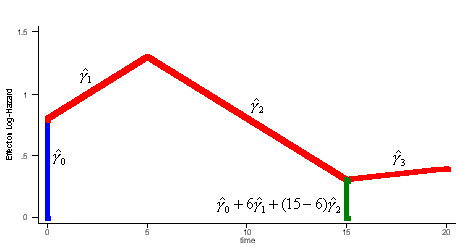Illustrative Effect on Log-Hazard
Consider the above figure. Suppose you estimated the effect of some continuous time variable (age, duration since leaving school, duration since wedding, etc.) as a duration spline with nodes at 6 and 15 years:
define spline Effect; nodes = 6 15; intercept;
hazard model; ...;
model = ... + durspline(origin=0, ref=Effect) + ...;
The estimated spline coefficient include an intercept,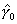, shown in blue, and three slopes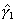,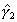, and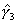. The significance of the variable's effect at the origin is easily determined from the significance of. But what about at other points in time? Is the effect significant at, say, time=15? We thus want to test whether the distance indicated in green,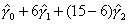, is significantly different from zero.

One approach is to derive the distribution of the test statistic: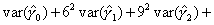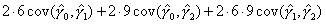. This requires that you know the variances and covariances of,, and. AML writes those out optionally, with the "option variance-covariance matrix". While not difficult, it involves a little algebra. An alternative approach applies a trick: redefine the spline such that the origin is at the point in time where you wish to test for significance, so that the intercept becomes the parameter of interest. In this case:

define spline NewEffect; nodes = -9 0; intercept;
hazard model; ...;
model = ... + durspline(origin=-15, ref=NewEffect) + ...;
Note that we relocated the nodes to be the same as before, but now measured relative to time=15. In the model specification, origin=-15, i.e., 15 years after the beginning of the hazard spell. The two models are equivalent. The estimated slopes will be identical to the original slopes,, and. The intercept will be equal to, and the problem reduces to a simple t-test.

This procedure does not generalize to "kick-in" splines (with "effect=right"), but it does readily generalize to splines that have non- zero origins and to tests at points that do not correspond to nodes. For example, to test whether the effect is significant at time=20:

define spline NewEffect; nodes = -14 -5; intercept;
hazard model; ...;
model = ... + durspline(origin=-20, ref=NewEffect) + ...;